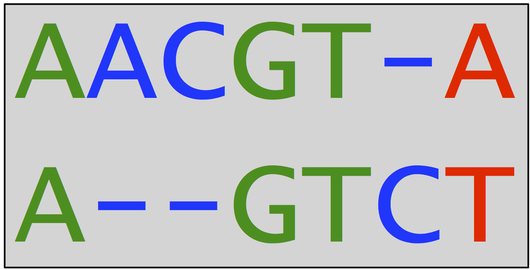# Glossary

## Augmented string

Given a string, its augmented string is formed by adding gap symbols to it. For the strings $s = \textrm{AACGTA}$ and $t = \textrm{AGTCT}$, we may add gap symbols to $s$ and $t$ to produce the augmented strings $\overline{s} = \textrm{AACGT-A}$ and $\overline{t} = \textrm{A--GTCT}$.

Assuming that two augmented strings have the same length, they may be compared by aligning them, which offers a scenario by which one string could have been transformed into another via the substitution, insertion, and deletion of symbols. We assume that gap symbols from the two augmented strings are not found in corresponding positions. Gap symbols in $\overline{s}$ are matched against symbols in $\overline{t}$ that were inserted into $s$; gap symbols in $\overline{t}$ are matched against symbols in $\overline{s}$ that were deleted from $s$.

For example, let us reconsider the augmented strings $\overline{s} = \textrm{AACGT-A}$ and $\overline{t} = \textrm{A--GTCT}$, which are illustrated below. The simplest way of inferring a collection of point mutations transforming $s$ into $t$ is to assume that the 'A' and 'C' from the second and third positions of $\overline{s}$ were deleted, a 'C' was inserted into the sixth position of $\overline{s}$, and the final symbol was changed from an 'A' to a 'T'. In the figure, we encode substitutions in red and insertions/deletions in blue; symbols that are fixed are colored green.